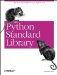# The copy Module

The copy module contains two functions that are used to copy objects, as shown in Example 1-64.

copy(object) => object creates a "shallow" copy of the given object. In this context, shallow means that the object itself is copied, but if the object is a container, the members will still refer to the original member objects.

Example 1-64. Using the copy Module to Copy Objects

```File: copy-example-1.py

import copy

a = [,,]
b = copy.copy(a)

print "before", "=>"
print a
print b

# modify original
a = 0
a = None

print "after", "=>"
print a
print b

before =>
[, , ]
[, , ]
after =>
[, None, ]
[, , ]```

You can also make shallow copies of lists using the [:] syntax (full slice), and you can make copies of dictionaries using the copy method.

In contrast, deepcopy(object) => object creates a "deep" copy of the given object, as shown in Example 1-65. If the object is a container, all members are copied as well, recursively.

Example 1-65. Using the copy Module to Copy Collections

```File: copy-example-2.py

import copy

a = [,,]
b = copy.deepcopy(a)

print "before", "=>"
print a
print b

# modify original
a = 0
a = None

print "after", "=>"
print a
print b

before =>
[, , ]
[, , ]
after =>
[, None, ]
[, , ]```Python Standard Library (Nutshell Handbooks) with
ISBN: 0596000960
EAN: 2147483647
Year: 2000
Pages: 252
Authors: Fredrik Lundh